SLUSA75B July   2010  – January 2020

PRODUCTION DATA.

1. Features
2. Applications
3. Description
1.     Device Images
4. Revision History
5. Description (continued)
6. Pin Configuration and Functions
7. Specifications
8. Detailed Description
1. 8.1 Overview
2. 8.2 Functional Block Diagram
3. 8.3 Feature Description
4. 8.4 Device Functional Modes
9. Application and Implementation
1. 9.1 Application Information
2. 9.2 Typical Application
1. 9.2.1 Design Requirements
2. 9.2.2 Detailed Design Procedure
3. 9.2.3 Application Curves
10. 10Power Supply Recommendations
11. 11Layout
12. 12Device and Documentation Support
13. 13Mechanical, Packaging, and Orderable Information

• RVA|16
• RVA|16

#### 9.2.2.4 Power MOSFETs Selection

Two external N-channel MOSFETs are used for a synchronous switching battery charger. The gate drivers are internally integrated into the IC with 6 V of gate drive voltage. 30 V or higher voltage rating MOSFETs are preferred for 20-V input voltage, and 40 V or higher rating MOSFETs are preferred for 20-V to 28-V input voltage.

Figure-of-merit (FOM) is usually used for selecting a proper MOSFET based on a tradeoff between conduction loss and switching loss. For a top-side MOSFET, FOM is defined as the product of the MOSFET's on-resistance, RDS(on), and the gate-to-drain charge, QGD. For a bottom-side MOSFET, FOM is defined as the product of the MOSFET's on-resistance, RDS(on), and the total gate charge, QG.

Equation 17.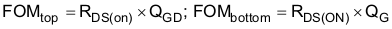The lower the FOM value, the lower the total power loss. Usually a lower RDS(on) has a higher cost with the same package size.

Top-side MOSFET loss includes conduction loss and switching loss. It is a function of duty cycle (D = VOUT/VIN), charging current (ICHG), the MOSFET's on-resistance RDS(on), input voltage (VIN), switching frequency (F), turnon time (ton) and turnoff time (toff):

Equation 18.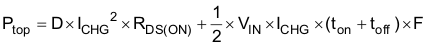The first item represents the conduction loss. Usually MOSFET RDS(ON) increases by 50% with 100°C junction temperature rise. The second term represents switching loss. The MOSFET turnon and turnoff times are given by:

Equation 19.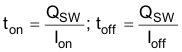where

• QSW is the switching charge,
• Ion is the turnon gate driving current,
• and Ioff is the turnoff gate driving current.

If the switching charge is not given in the MOSFET datasheet, it can be estimated by gate-to-drain charge (QGD) and gate-to-source charge (QGS):

Equation 20.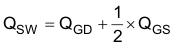The gate driving current total can be estimated by the REGN voltage (VREGN), MOSFET plateau voltage (VPLT), total turnon gate resistance (Ron), and turnoff gate resistance (Roff) of the gate driver:

Equation 21.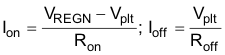The conduction loss of the bottom-side MOSFET is calculated in Equation 22 when it operates in synchronous continuous conduction mode:

Equation 22.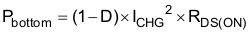If the SRP-SRN voltage decreases below 5 mV (the charger is also forced into non-synchronous mode when the average SRP-SRN voltage is lower than 1.25 mV), the low-side FET is turned off for the remainder of the switching cycle to prevent negative inductor current.

As a result, all of the freewheeling current goes through the body diode of the bottom-side MOSFET. The maximum charging current in non-synchronous mode can be up to 0.9 A (0.5 A typical) for a 10-mΩ charging current sensing resistor, considering the IC tolerance. Choose a bottom-side MOSFET with either an internal Schottky or body diode capable of carrying the maximum non-synchronous mode charging current.

MOSFET gate driver power loss contributes to dominant losses on the controller IC, when the buck converter is switching. Choosing a MOSFET with a small Qg_total reduces power loss to avoid thermal shutdown.

Equation 23.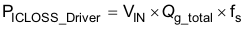where

• Qg_total is the total gate charge for both the upper and lower MOSFETs at 6V VREGN.